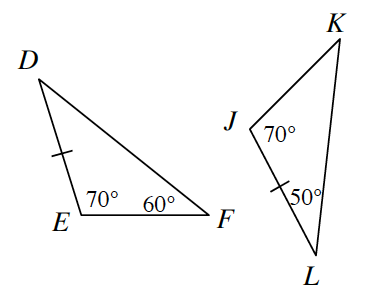### Home > INT2 > Chapter 2 > Lesson 2.1.4 > Problem2-42

2-42.

Examine the two triangles at right.

1. Are the triangles congruent? Justify your conclusion. If they are congruent, complete the congruence statement $ΔDEF$_____ .

Use the Triangle Angle Sum Theorem to help find the missing angles, then determine whether or not the triangles are similar.

2. What sequence of transformation(s) will map $ΔDEF$ onto $ΔJKL$?

Reflection and rotation.